# A brief exploration of mathematical modelling

By
Nicholas Munro

This is an edited version of the winning article in the ages 11-15 category of the 2017 BSHM schools writing competition. You can also read the winning article in the 16-19 category.

Mathematical models describe a system using a set of mathematical concepts and functions. The system in question could be anything from the minute to the massive; from the physics behind an insect skimming across the water's surface to the orbits of exoplanets. The key concepts of how these ideas are translated into equations remain the same.The merging of water droplets can be understood using mathematical models.

As one fluid dynamics PhD researcher who uses models daily puts it, "Mathematical modelling tries to explain the real world around us by reducing complicated and messy things down to simple ideas. The aim is to show that those simple ideas are enough to give the complicated effect that you're trying to explain, because then you know that you've reduced the problem down to what was really important."

In building models we need to compromise: most interacting systems are far too complex to be described in their entirety, hence it is best to identify the pivotal concepts of a system to include in a model, and exclude the rest.

The inherent advantage of mathematically modelling is that it allows for precise and well-defined manipulation using proven mathematical principles. This manipulation is extremely useful for performing hypothetical tests or constructing simulations. The knowledge that humans have accumulated of the intimate workings of mathematics helps to understand the effects described by the models we create. In addition, computers can be used to perform numerical calculations, enhancing the capacity for simulating outcomes.

### What are mathematical models used for?

In the first instance, models are used to extend scientific understanding. By modelling what is already known, some of what is currently unknown may become deductible. PhD student James Munro's research aims to understand why drops of liquids coalesce as quickly as they do. Certain elements of this problem are already known. "We know how liquids move, not just for drops joining, but the general sort of rules that liquids should obey," says Munro. "These rules are mathematical equations of the speed of the liquid."

Munro explains that these equations, once they have been simplified to the meaningful principles, are then used to model to experiments in which droplets join, and solved to find a value for the speed of the liquid. "The aim is to explain the experiments, and the method is to solve the simplest possible mathematical equations to get a useful answer." These useful answers contribute to understanding of the original problem.

Models are also exploited to study potential effects of changes to a system. For example, legislators and governments might want to assess the impact of changes to laws and regulations on a range of indicators to help them make decisions. This will help to plan for, and respond to, crises such as epidemics.

### How are mathematical models constructed?The spread of infectious diseases can be understood using the SIR model.

Let's use the spread of infectious diseases as an example. Mathematical models of the spread help legislators and health officials to take precautions against outbreaks and react to them appropriately.

We will look at the basic SIR (Susceptible, Infected, Recovered) model of an infectious disease. It assumes a homogenous population of a fixed number of people. The first step of building a model is to state the basic assumptions that are made about the way the system that is being modelled operates. In the case of the SIR model these are:

1. Those who are infected can spread the disease on to those who are susceptible.
2. Those who are infected recover over a period of time and become immune.

We will assume that time passes in discrete steps: there is a time 0, a time 1, a time 2, and so on. We will write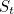for the proportion of susceptible people at time,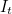for the proportion of infected people at time, and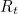for the proportion of recovered people at time.

We assume that the disease spreads through a series of events. The first event is random contact between an infected person and a susceptible person leading to a new infection. We will write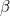for the probability of an infected person infecting a susceptible one. The proportion of newly infected people must be subtracted from the susceptible class and added to the infected class. We can express this in equations relating the proportions of people in each class at timeto the proportion at the next time step along,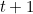: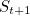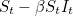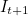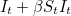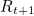The next event that is assumed to happen is that people who are infected eventually recover with probability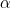. These people are subtracted from the infected group and added to the recovered group: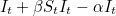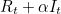A central feature of this model is that the size of the population will always remain the same. For this to make sense in a real, human population, we must assume, either that birth and death rates are equal, or that the people who have died are included in the recovered class and no new births are occurring. These unrealistic assumptions highlight the short fallings of this basic model, but they can be addressed in a more sophisticated model.

There is another important feature we should notice about the model. The parametersandcombine several factors that a more sophisticated model might treat separately. The chance of a susceptible person becoming infected,, depends on a range of factors, such as the quality of sanitation and the density of the population. Similarly the chance of recovery,, depends the quality of healthcare in a country. Investigating and calculating how to quantify such factors and representing them as a numerical parameter is a key part of constructing and interpreting models. (To read about the SIR model in more detail see The mathematics of diseases.)

### A word of cautionWill maths give you the mortgage?

Models today have huge influence on society. They are used in a wide range of areas, from epidemiology to cancer research, and from engineering to economics (you can find out more here). But improper use and ignorant model design have in the past lead to major disturbances. A well-documented example of this is the 2008 global financial crash.

Financial models are used in markets to estimate the value of stocks and asses the risk associated with them and how those risks can be controlled. The root cause of the 2008 crash was the sudden realisation that the predicted values of so-called CDOs (bundles of investments that included sub-prime mortgages) were far below the values that models had led traders to believe (see here to find out more).

The problem, argue Emanuel Derman and Paul Wilmott in their 2009 Financial Modellers' Manifesto, was that some of the models used at the time of the crash reduced all the uncertainty involved in calculating investment value to a single parameter. This was done to make the model useable and to conceal the considerable complexity of the movement of thousands of mortgages.

Unlike maths or physics, there are no fundamental rules governing human behaviour. Financial models should make no pretence to encapsulate all an economy's complexity. Doing that is turning a blind eye to the deficiencies of the model's assumptions — and it's what happened in the sub-prime mortgage crash, when the true risks involved were swept under the rug.

Derman and Wilmott go on to say that the most understandable financial models are superior; ones that postulate a vision of the system that, though idealised, is explicit in its oversights and allows a skilled human trader to account for the excluded parameters.

The Financial Modeller's Manifesto ends with the modeller's hippocratic oath, which offers a set of standards for more ethical and transparent modelling.

"I will remember that I didn't make the world, and it doesn't satisfy my equations."